# Test: Amplifiers

## 10 Questions MCQ Test RRB JE for Electrical Engineering | Test: Amplifiers

Description
Attempt Test: Amplifiers | 10 questions in 30 minutes | Mock test for Railways preparation | Free important questions MCQ to study RRB JE for Electrical Engineering for Railways Exam | Download free PDF with solutions
QUESTION: 1

Solution:
QUESTION: 2

### Consider the circuit of fig. The transistor parameters are  β = 120 and VA = ∞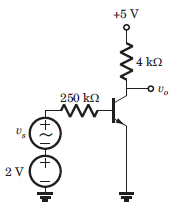Que: The hybrid-π parameter values of gm ,rπ  and ro are

Solution: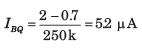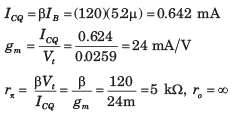QUESTION: 3

### Consider the circuit of fig. The transistor parameters are  β = 120 and VA = ∞Que: The small signal votlage gain Av = vo/vs

Solution: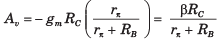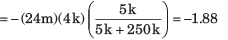QUESTION: 4

The nominal quiescent collector current of a transistor is 1.2 mA. If the range of β for this transistor is 80 ≤ β ≤ 120 and if the quiescent collector current changes by +-10 percent, the range in value for rπ is

Solution: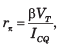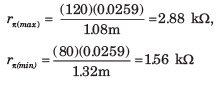QUESTION: 5

Consider the circuit in fig. The transistor parameter are β = 100 and VA = ∞.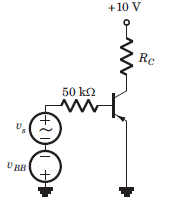If Q-point is in the center of the load line and ICQ = 0.5 mA, the values of VBB and RC are

Solution: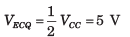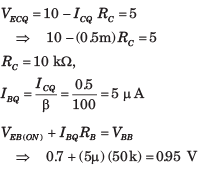QUESTION: 6

Consider the common Base amplifier shown in fig. The parameters are β = 100, gm = 2 mS and ro = 250 kΩ. Find the Thevenin equivalent faced by load resistance RL .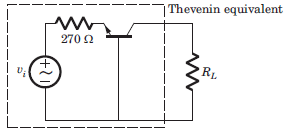Que: The Thevenin voltage vTH is

Solution:

The equivalent circuit is shown below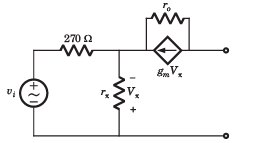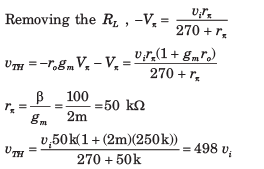QUESTION: 7

Consider the common Base amplifier shown in fig. The parameters are gm = 2 mS and ro = 250 kΩ. Find the Thevenin equivalent faced by load resistance RL .Que: The Thevenin equivalent resistance RTH is

Solution:

The equivalent small-signal circuit is shown in fig.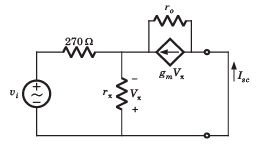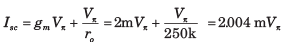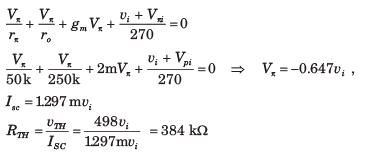QUESTION: 8

The common-base amplifier is drawn as a two-port in fig. The parameters are β =100, gm = 3 mS, and ro =  800 kΩ.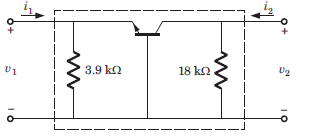Que: The h-parameter h21 is

Solution:

The equivalent small-signal circuit is shown in fig.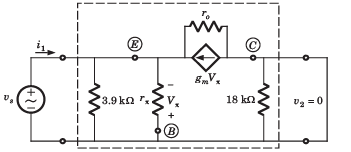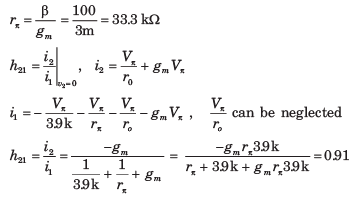QUESTION: 9

The common-base amplifier is drawn as a two-port in fig. The parameters are β =100, gm = 3 mS, and ro =  800 kΩ.Que: The h-parameter h12 is

Solution: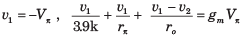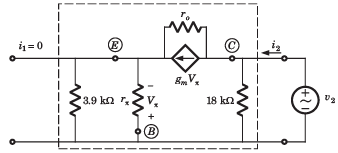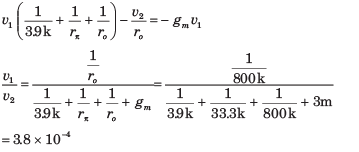QUESTION: 10

Consider the source-follower circuit in fig. The values of parameter are gm = 2 mS and ro = 100 kΩ.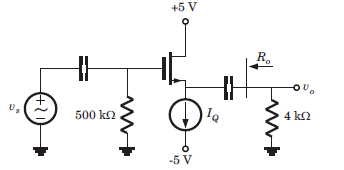Que: The voltage gain Av is

Solution:

The small-signal equivalent circuit is shown in fig.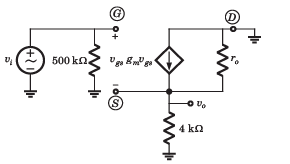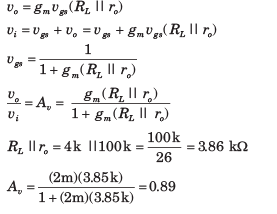Use Code STAYHOME200 and get INR 200 additional OFF Use Coupon Code